CSC 378: Data Structures and Algorithm Analysis

Buildheap, with Analysis

[CLR 7.3]

# Building a Heap

With A[1...n] initially randomly filled, execute: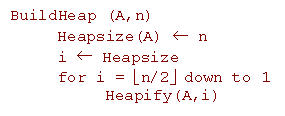Look at it as a tree: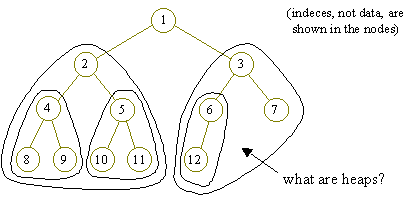In the example,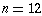, so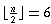and the code executes:

heapify(A,6)
heapify(A,5)
heapify(A,4)
heapify(A,3)
heapify(A,2)
heapify(A,1)

Ordering is important!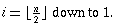# Analysis of BuildHeap

The easy way: Each Heapify takes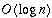time, and there are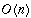calls to Heapify, so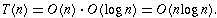But a more detailed analysis yields a tighter bound. Heapify(i) takes time proportional to the height of node i. So count the individual steps: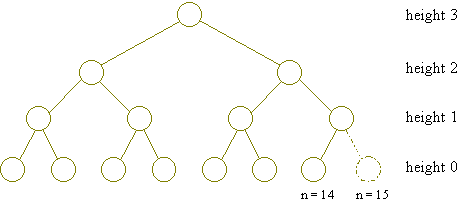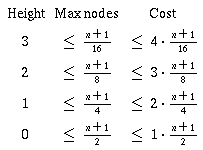The number of nodes at height i is at most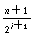.

The cost of Heapify at height i is at most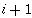per node.

So the cost of BuildHeap is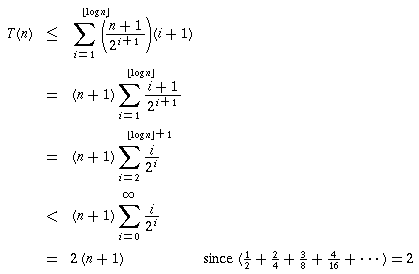Therefore,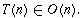# Heapsort

Here's the Heapsort procedure, which reorganizes the array so that A, A, ... A[n] are in increasing order:

```HeapSort(A,n) BuildHeap(A,n) for i=0 to n-1 A[n-i] = ExtractMax(A)```

Since BuildHeap takestime and each of the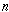ExtractMaxs takestime, the array is sorted in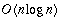time.

Back to the lecture index